# Indifference curves - prices and demand

## Indifference curves – prices and demand

### Identifying consumer equilibrium

If we combine data for the budget lines and indifference curves we can establish when a consumer is in equilibrium and maximising their utility.

We can use the data from the sweaters and socks example.

Reveal figures for budget line and indifference curve

Graphically, equilibrium will be where the budget line is at tangent to the relevant indifference curve, as shown below: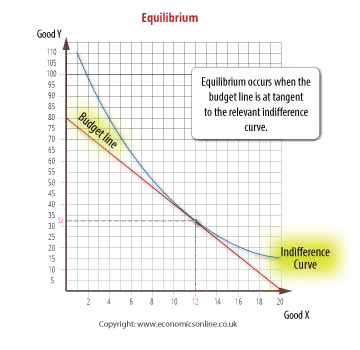In the sweaters-socks example, with income at 400, the price of x (sweaters) at 20, and the price of y (socks) at 5, equilibrium will be at 32y and 12x.

This is the only point at which the gradient of the budget line and indifference curve are identical.

#### The equi-marginal principle

We saw earlier that the MRS (the gradient of the indifference curve) is Δy/Δx. The MRS can also be expressed in terms of marginal utility – indeed, the MRS is the negative ratio of the marginal utility of an extra unit of x (sweaters) to the marginal utility of an extra unit of y (the socks), hence:

MRS = Δyx = (-) MUx/MUy.

When in equilibrium, the gradient of the budget line (the ratio of prices Px/Py) will equal the gradient of the indifference curve, which when expressed in terms of marginal utility, is (-) MUx/MUy.  Hence, we have:

(-) MUx/MUy = (-) Px/Py, which can be re-written as:

MUx/Px = MUy/Py.

Hence, at equilibrium the ratio of MU to prices is identical for all goods and services bought, meaning that each marginal unit of currency spent yields the same utility – referred to as the ‘equi-marginal principle’. When this condition is met the individual is maximising their total utility.

### The price-consumption line

Indifference analysis can help us understand how demand responds to changes in price.

If we change the price of one good, say good x, the budget line will rotate, fixed at the y-axis, and a new point of tangent between the new budget line and new indifference curve will be found.

If we keep altering price, the sequence of new equilibrium points provides us with a price-consumption line, as shown below.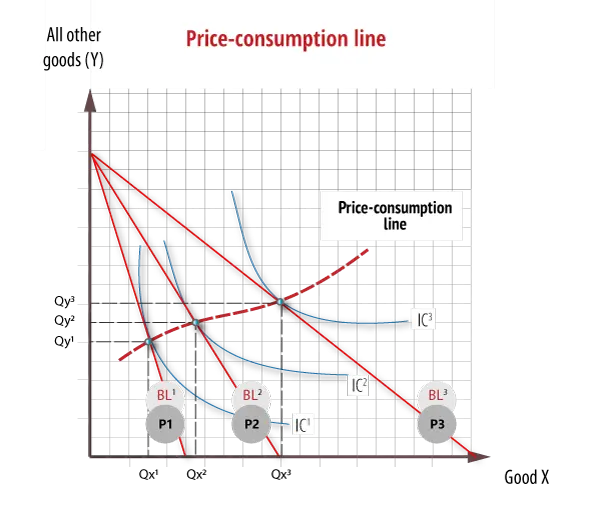### Deriving a demand curve

Indifference curves can be used to derive a demand curve. If we assume a basket of only two types of good, and hold income constant, we can derive a demand curve which shows the quantity demanded for a good at different prices.

The prices change in the indifference diagram can be converted into a standard demand diagram, as shown below.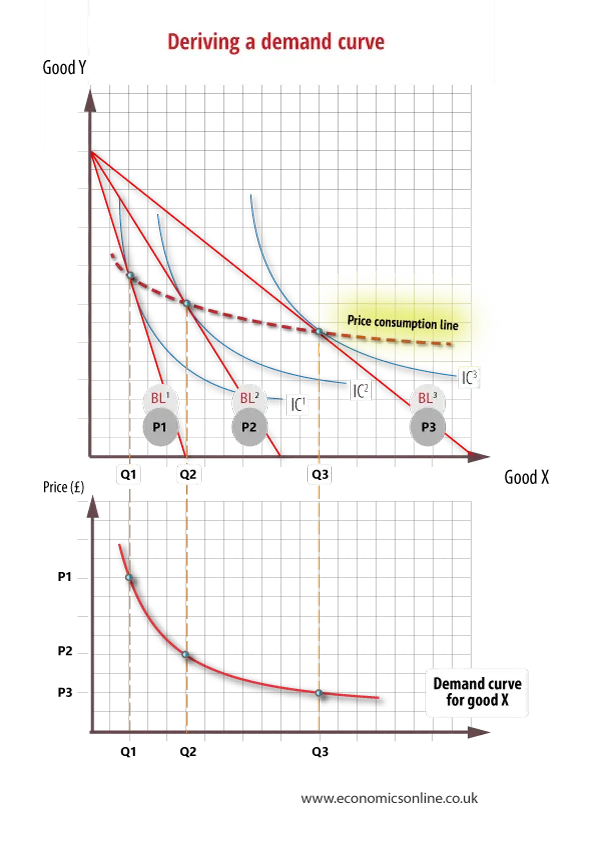### Distinguishing the income and substitution effect

Whenever price changes it creates two effects, the income effect – which explains how a change in price affects real income – and the substitution effect, which explains how changes in price alter the attractiveness of one good in terms of alternative ways of spending money.

In terms of indifference curve analysis, the substitution effect is derived from the gradient of the budget line when it pivots inwards or outwards – inwards for a price increase, and outwards for a price decrease.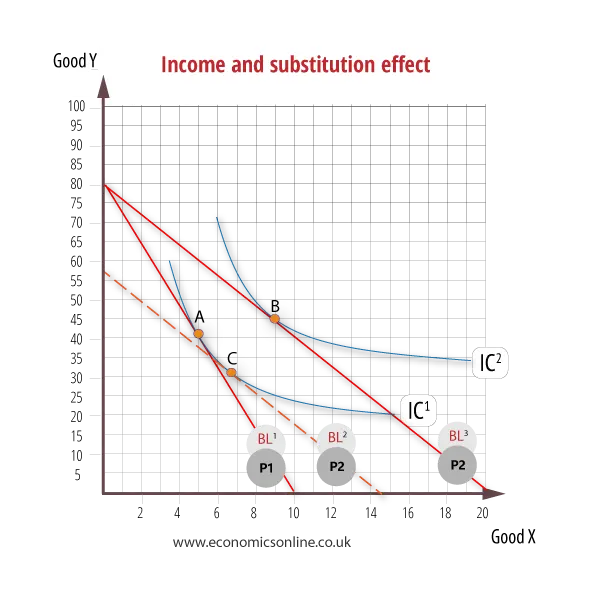In the above diagram, which relates to the sweaters/socks example, the price reduction from £40 to £20 for good x rotates the budget line outwards at the x-axis. The overall effect of the price reduction is to increase quantity demanded from 5 to 9 units of x.

To find the breakdown of this in terms of the income and substitution effect we create an additional budget line parallel to the new budget line for the lower price. The quantity relating to the substitution effect is the movement from BL1 to BL2 (tangent A to C) – from 5 to 7 units of x.

The second effect, relates to the income effect, and this is shown from the pivoted budget line (BL2) to the new budget line for the price reduction, at BL3.   This is shown as the movement from the tangent point at C to B, which in this case is the increase from 7 to 9 units of x.

### Showing price elasticity of demand

Price elasticity of demand (PED) can be shown with the help of a price-consumption line. The most convenient way to show it is to have ‘one’ good on the x-axis, and ‘all other goods’ bundled together on the on the y-axis. In this case we can create hypothetical indifference curves for choices which involve one good, (good x), and all other available goods (bundle y).

PED is shown by the formula:

PED%∆Qd= (-)%∆P

We can apply the formula to the sweaters-socks example, for the price range A (from £80 to £40) and B (from £40 to £20) as shown in the graph.Hence, as the budget line rotates to the right, and the price falls, the value of PED also falls. However, in both cases shown, PED is greater than 1, and is elastic (PED over the price range A = (-) 3.0, and over price range B = (-) 2.8. An inelastic response of less than 1 would require a much steeper price consumption line, as shown below.#### Giffen goods

A Giffen* good is one that does not follow the standard rule regarding how price changes affect the quantity demanded. Typically, price and quantity are inversely related, but, with a Giffen good, price and quantity demanded are positively related.

In the diagram below we see that as the price rises, with the budget line rotating to the left, more is demanded, and as the price falls and the budget line rotates to the right, less is demanded.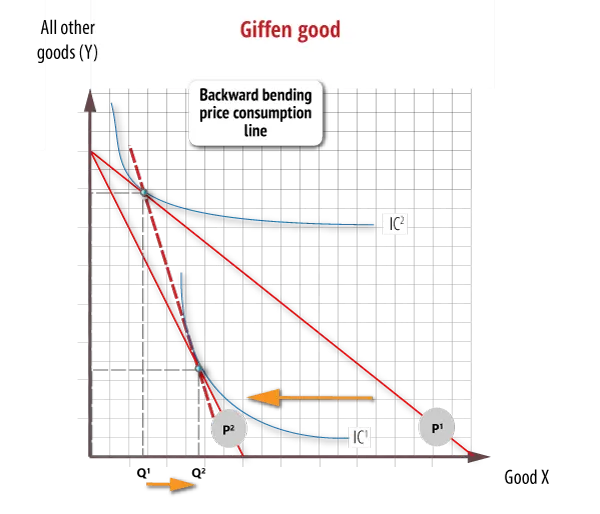With a Giffen good, the income effect outweighs the substitution effect.

*Named after Sir Robert Giffen

#### Price consumption line showing different elasticities

Indifference curves can also be used to show variations in the PED for one good, where it is possible to have a very elastic response over one price range and a very inelastic one over another, as shown below.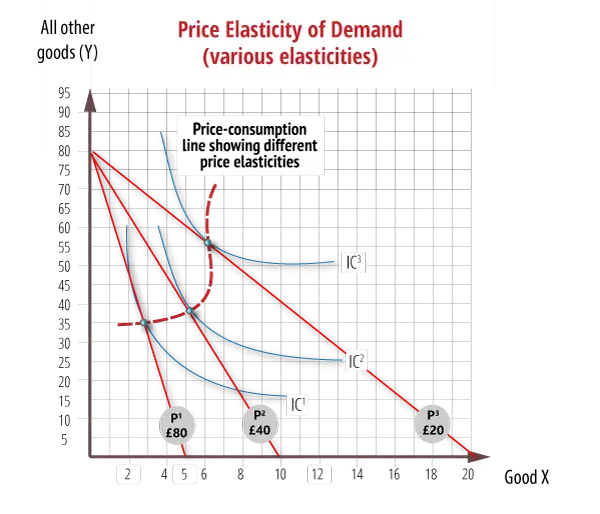Go to further applications of indiference curves:

Income, normal and inferior goods